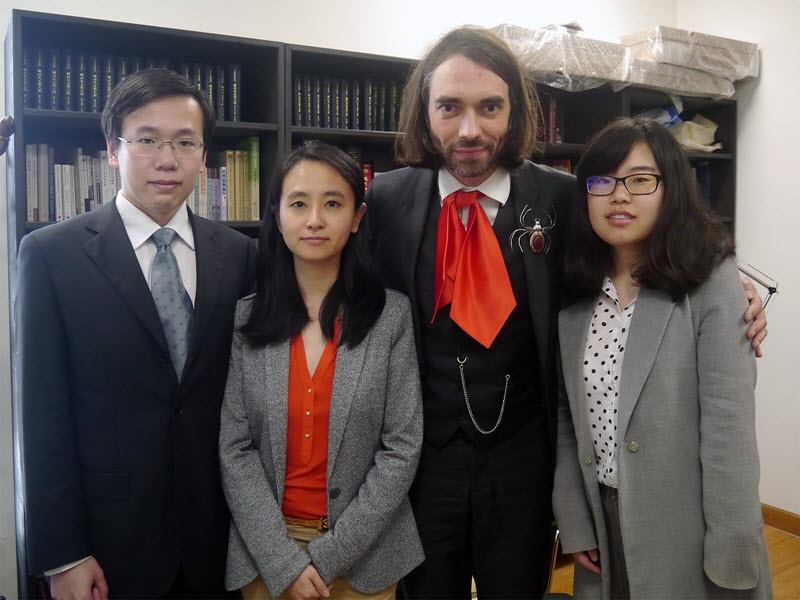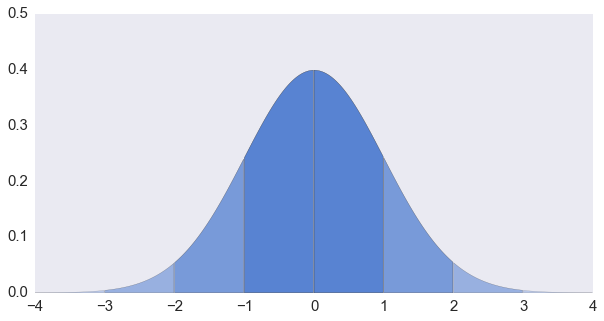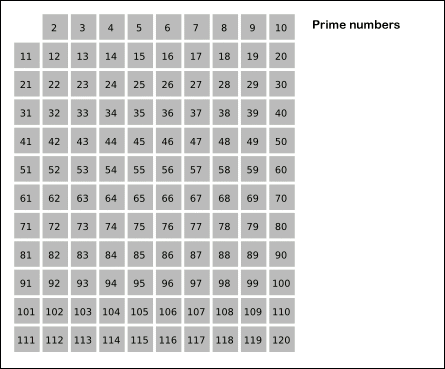# &#65279 引发奇怪的空白

Visual Studio 在保存 UTF-8 文件时默认是 “with signature” 的，这就会保存 BOM 字符。

# 塞德里克·维拉尼教授访谈录1. Try restarting the computer by selecting “last known good configuration” in the boot options.
2. If the restart does not fix the problem, the registry damage is too extensive. You must reinstall the OS or use the Emergency Repair Disk (ERD) that you previously created by using the Windows Backup tool.

# 正态分布随机数的生成 (2)

## 接受—拒绝法# VMware Player 升级 / 卸载报错

The MSI '' failed

# 正态分布随机数的生成 (1)

$$f(x \; | \; \mu, \sigma) = \frac{1}{\sigma\sqrt{2\pi} } \; e^{ -\frac{(x-\mu)^2}{2\sigma^2} }$$# 蓄水池抽样浅说 (3)

#### 加个权吧

$$P_i = \frac{w_i}{w_1 + w_2 + \cdots + w_n}$$

$$P_n(1) = \frac{w_n}{w_1 + w_2 + \cdots + w_n}$$

$$P_{n-1}(2) = \frac{w_{n-1}}{w_1 + w_2 + \cdots + w_{n-1}}$$

$$P(S) = \prod_{i=1}^n \frac{w_i}{w_1+w_2+\cdots w_i}$$

# 蓄水池抽样浅说 (2)

#### 跳跳跳！—— Algorithm X

• 确定该跳过多少个元素 $S(k, n)$
• 跳过 $S(k, n)$ 个元素
• 从前 $k$ 个元素中随机产生一个要替换的元素，用下一个元素替换

# 蓄水池抽样浅说 (1)# 素数求和的动态规划方法